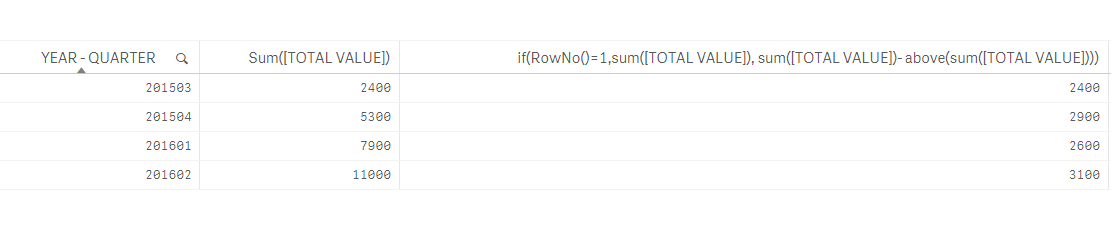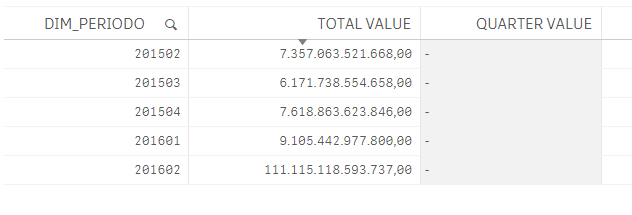# New to Qlik Sense

Discussion board where members can get started with Qlik Sense.

Announcements
BI & Data Trends 2021. Discover the top 10 trends emerging in today. Join us on Dec. 8th REGISTER
cancel
Showing results for
Did you mean:
HighlightedContributor III

## Get quarter value through accumulated value

Hello community, how are you?

The following problem occurred to me. I have a base for each quarter, which I am concatenating as they are loaded until a single table remains.

The totals of each quarterly amount are accumulated so that in order to obtain the value of the amount for that quarter I must calculate it.

To do this assemble the following table, so that my need can be understood.

YEAR - QUARTERTOTAL VALUEVALUE QUARTEREXPRESSION
20150324002400201503
20150453002900201504 - 201503
20160179002600201601 - 201504
201602110003100201602 - 201601

In the first column is the period that specifies the YEAR and the QUARTER. In the second column, the value of the accumulated amount for each quarter. Here I make a couple of clarifications: when the quarter is 2 (for example, 201602) the accumulated is also the annual accumulated. The other point to note is that the first quarter, that is, the quarter that only accumulates "its" quarter is 3 (for example, 201503). The remaining two columns represent the value that should be the amount of each quarter and how it is calculated.

The indicators that I must develop must show the amounts of the current and (and selected) quarter and the previous quarter and year. For this, I have on the dashboard a selector of YEAR and a QUARTER.

The expression I am using, is the following but I am given 0.

= sum(DIM_TOTAL_VALUE) -

sum({\$ < DIM_YEAR={\$(=DIM_YEAR_PREV)}, DIM_QUARTER={\$(=DIM_QUARTER_PREV)} > } DIM_TOTAL_VALUE)

Does anyone have any idea how to replicate the table I need?

Regards!

1 Solution

Accepted Solutions
HighlightedPartner

You have the accumulated total and want to have the value in each quarter?

If so, do as follow:

if(RowNo()=1,sum([TOTAL VALUE]), sum([TOTAL VALUE])- above(sum([TOTAL VALUE])))5 Replies
HighlightedMaster

Hi Martin,

Example of table in attached file.

Regards,

Andrey

HighlightedPartner

If you do have a value quarter for each quarter and you want  a table for example in which you'll have:

as a dimension: Quarter

as columns:

1) value of each quarter : sum(Value)

2) accumulated value   : rangesum(above( sum(Value),0,rowno())

HighlightedContributor III

Hi Andrey, thanks for your response and example.

Unfortunately it is not what I need. The table with which I count, among many fields is the following:

YEAR - QUARTERTOTAL VALUE
2015032400
2015045300
2016017900
20160211000

Through this table I have to obtain the quarterly values ​​(Column QUARTER) that are obtained from the expression (Column EXPRESSION).

When I want to replicate what happened to Andrey, I get the following and all I did was modify the name of the dimension:Always the quarter ending in '03' (Example: 201403,201503, 201603, etc) will have the same value for TOTAL VALUE as for VALUE QUARTER. If the first record is 201504, you should not apply the RowNo () = 1.

I appreciate your response and predisposition to help me solve this.

Regards!

HighlightedPartner

You have the accumulated total and want to have the value in each quarter?

If so, do as follow:

if(RowNo()=1,sum([TOTAL VALUE]), sum([TOTAL VALUE])- above(sum([TOTAL VALUE])))HighlightedContributor III

Excellent Omar! There I was able to get the results I needed!
Thank you very much for helping Omar and Andrey.
Regards!